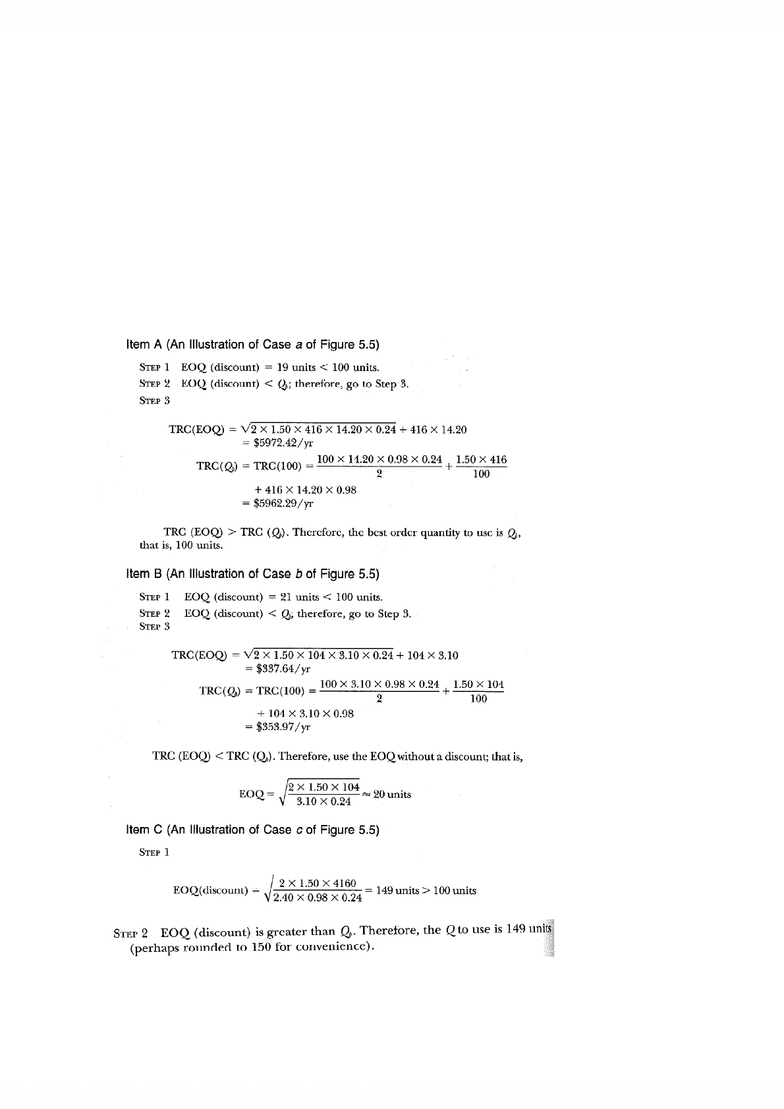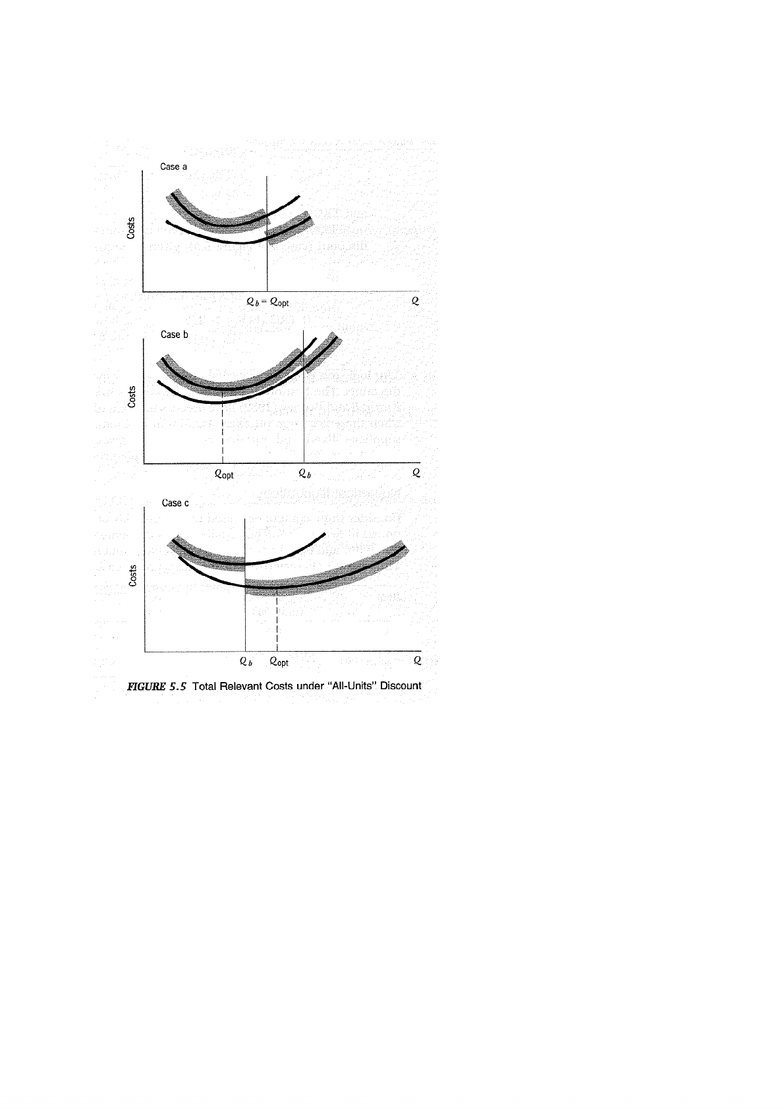# MSCI432 Lecture Notes - Carrying Cost, Msci, Safety Stock

99 views7 pagesMSCI 432/633: Production and Service Operations Management, Winter 2010
MSCI 432.001/633: Assignment # 3; MSCI 432.002: Assignment # 2
Q1. Discounting schemes.
a. Find the optimal ordering quantities for each of the items and the corresponding annual total cost.
[3 marks X3]
graphically. [2 marks]
Unlock document

This preview shows pages 1-2 of the document.
Unlock all 7 pages and 3 million more documents.Q2. Limit on shelf life.
Simply find T
EOQ
=EOQ/ߣ and if T
EOQ
>SL then Q
SL
=SL*λ
Q3. Uncertain demand.
a. Assume that the firm has centralized all inventories in a single warehouse and that the probability
of stocking out in a cycle can still be no more than 5%. Ideally, how much average inventory can
the company now expect to hold, and at what cost? In this case, how long will a unit spend in the
warehouse before being sold? [5 marks]
Unlock document

This preview shows pages 1-2 of the document.
Unlock all 7 pages and 3 million more documents.

## Document Summary

Msci 432/633: production and service operations management, winter 2010. Msci 432. 001/633: assignment # 3; msci 432. 002: assignment # 2. Discounting schemes: find the optimal ordering quantities for each of the items and the corresponding annual total cost. Simply find teoq=eoq/ and if teoq>sl then qsl=sl* . Uncertain demand: assume that the firm has centralized all inventories in a single warehouse and that the probability of stocking out in a cycle can still be no more than 5%. Solution: (since pipeline inventory is not influenced, we do not include it in the calculations below. ) (a) To determine the optimal order quantity, we will use the eoq formula. H = * 25%/yr = . 5/yr, d = 10,000 /wk, (i. e. , annual demand, d = 500,000/yr), and s = Average inventory at each warehouse (i) = q/2 + ss = (20,000/2) + 3,300 = 13,300 units. Average inventory holding cost per warehouse = h*i = . 5*13,300 = ,250.

# Get access

\$10 USD/m
Billed \$120 USD annually
Homework Help
Class Notes
Textbook Notes